# RS Aggarwal Solutions for Class 10 Maths Chapter 3 Linear Equations in Two Variables Exercise 3D Page number 122

R S Aggarwal Solutions for Class 10 Maths Chapter 3 Linear Equations in Two Variables, contains solutions for exercise 3d questions. These solutions are prepared by subject matter experts at BYJU’S, explaining about conditions for the solvability of linear equations. Students can download the R S Aggarwal Solutions of Class 10  and score well in their exams.

## Download PDF of RS Aggarwal Solutions for Class 10 Maths Chapter 3 Linear Equations in Two Variables Exercise 3D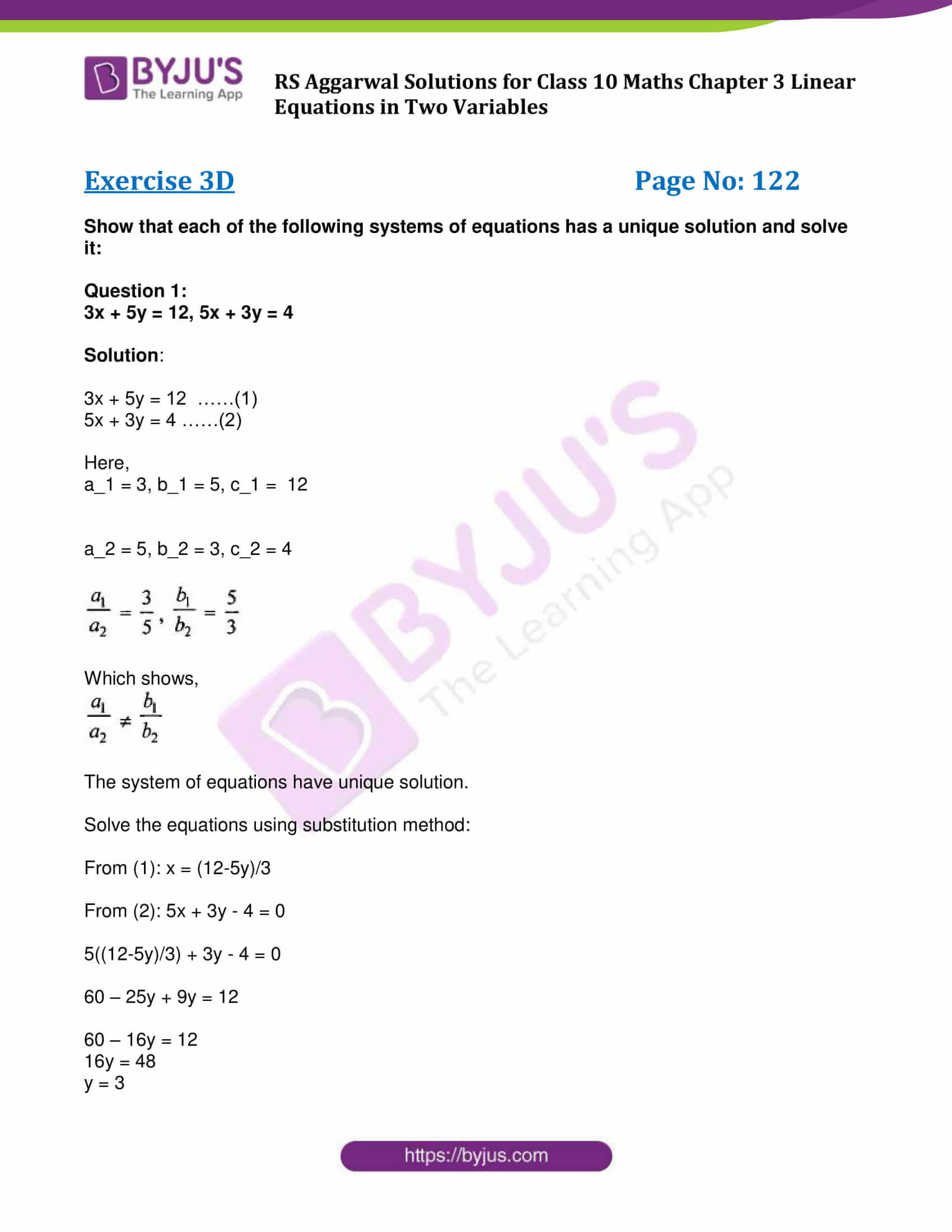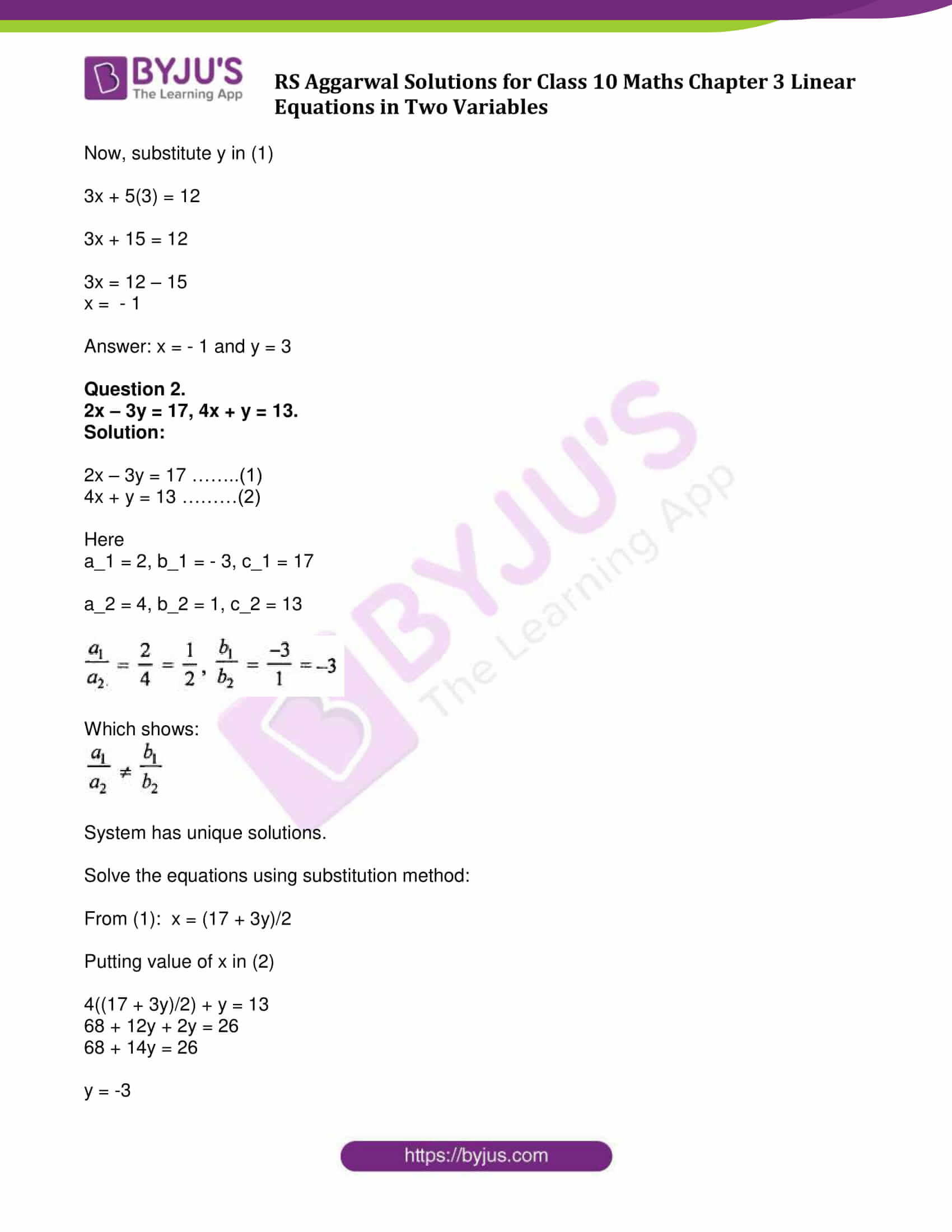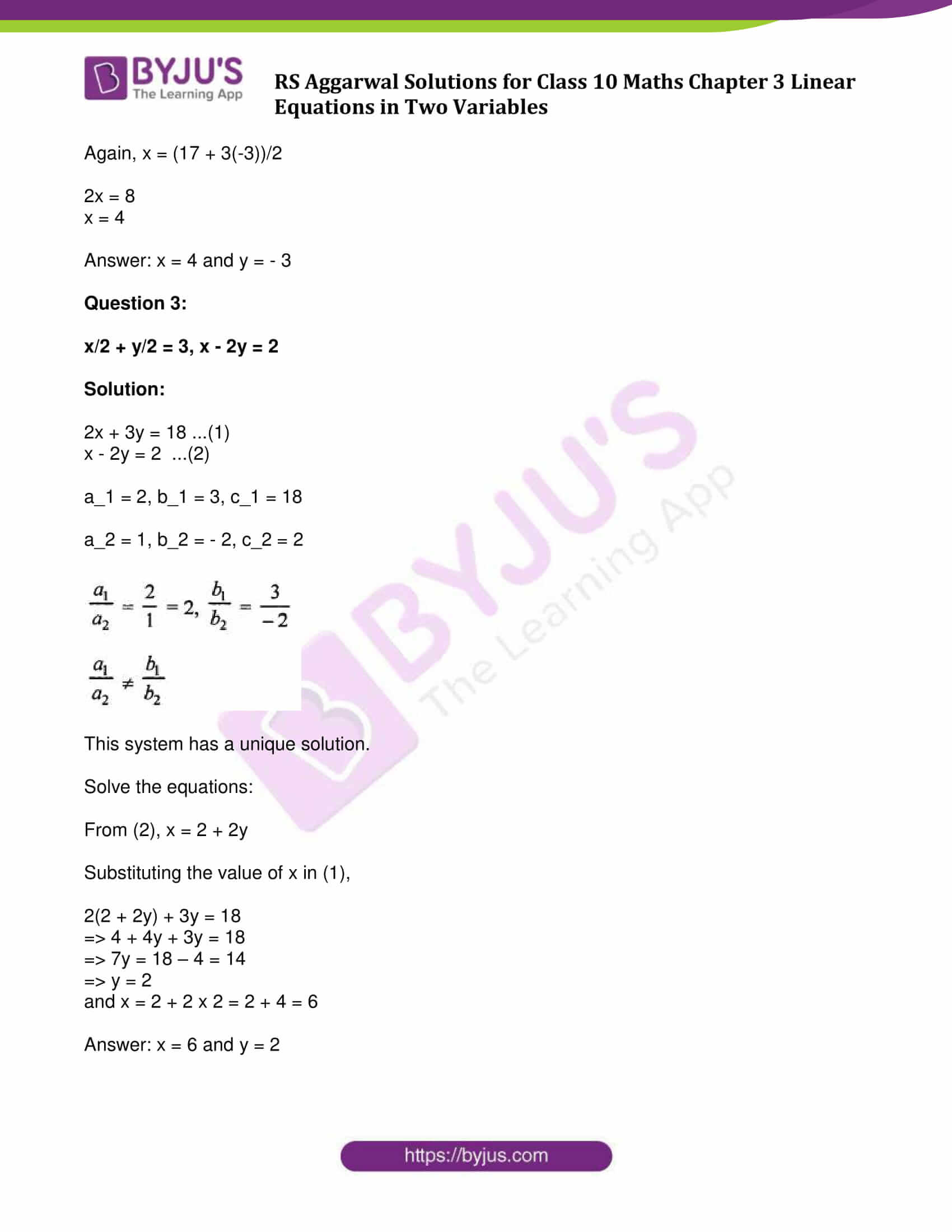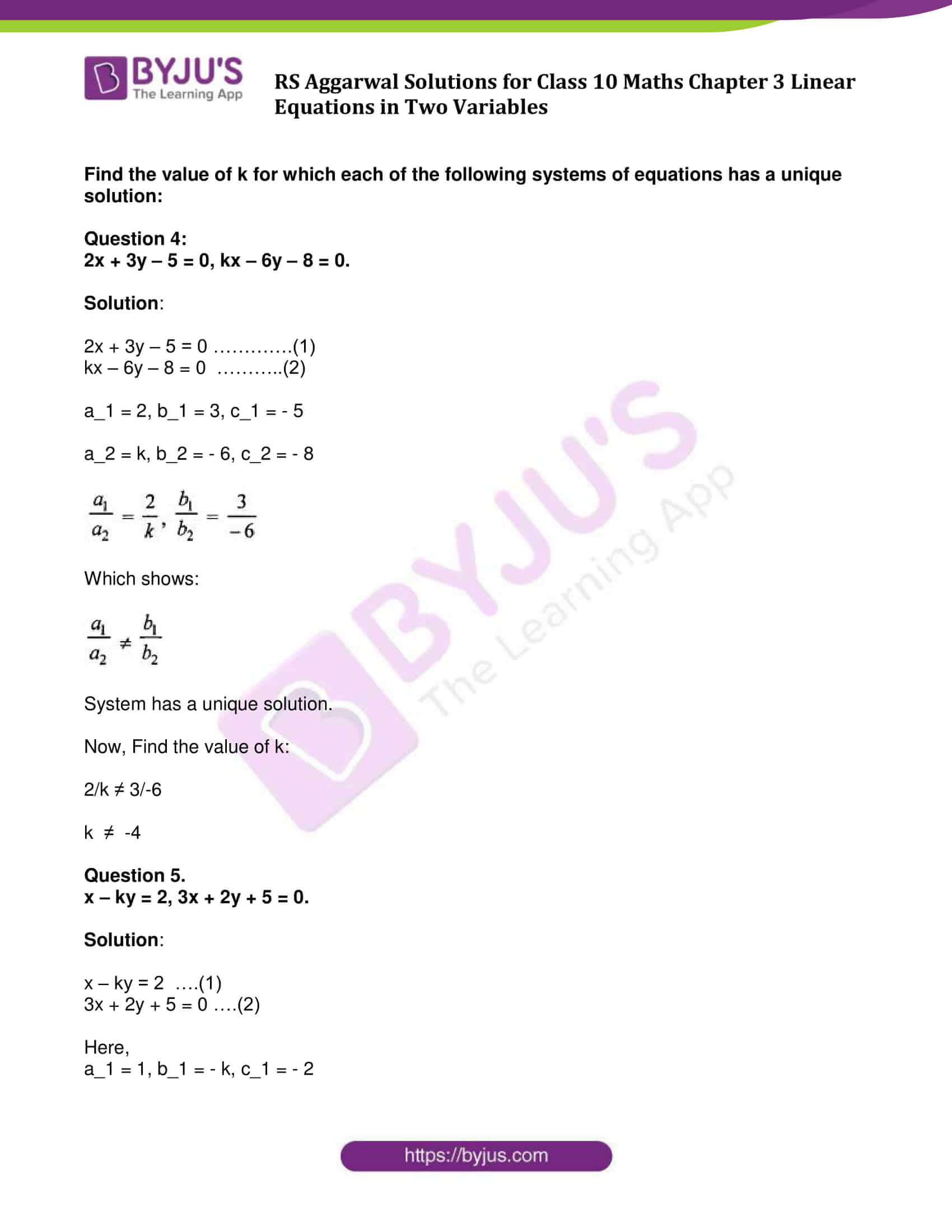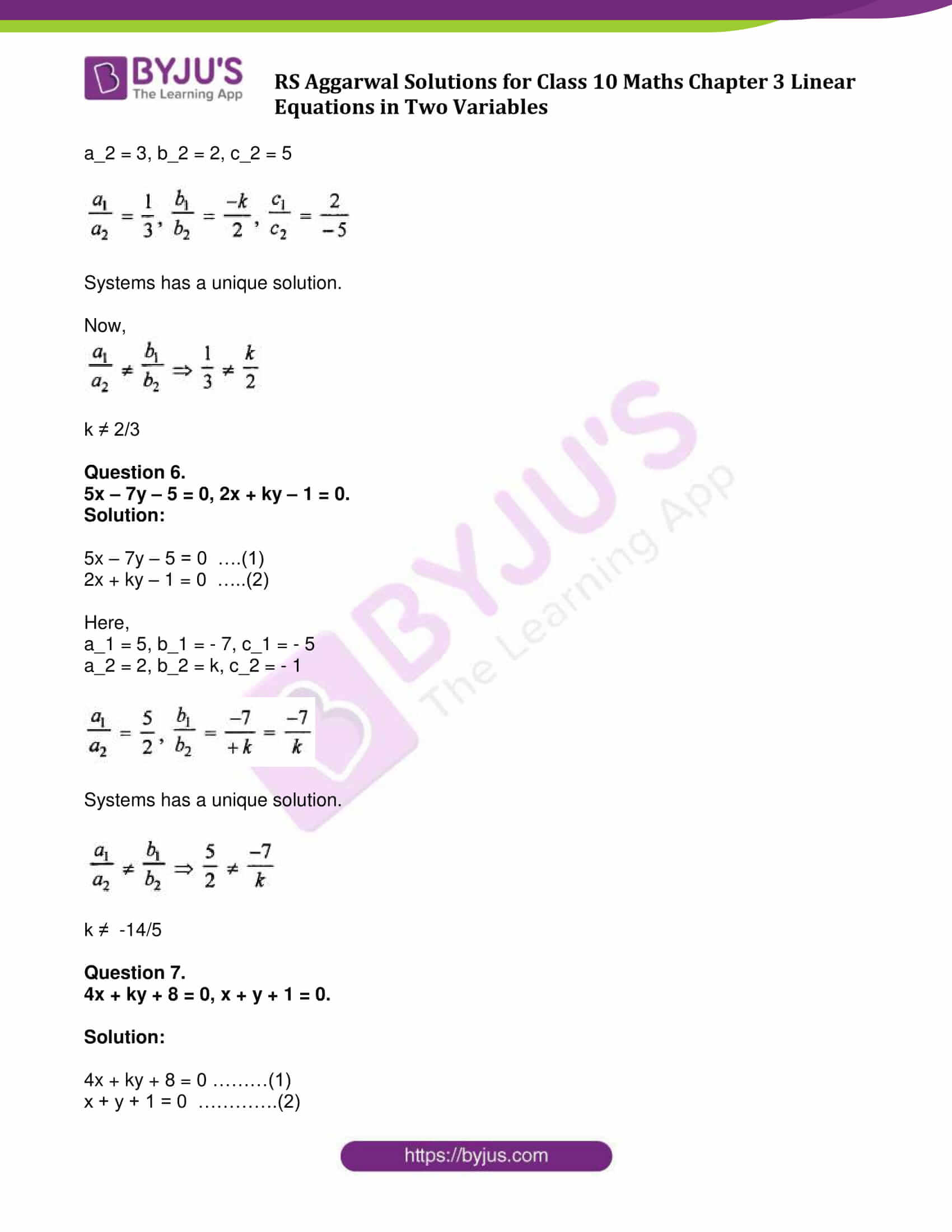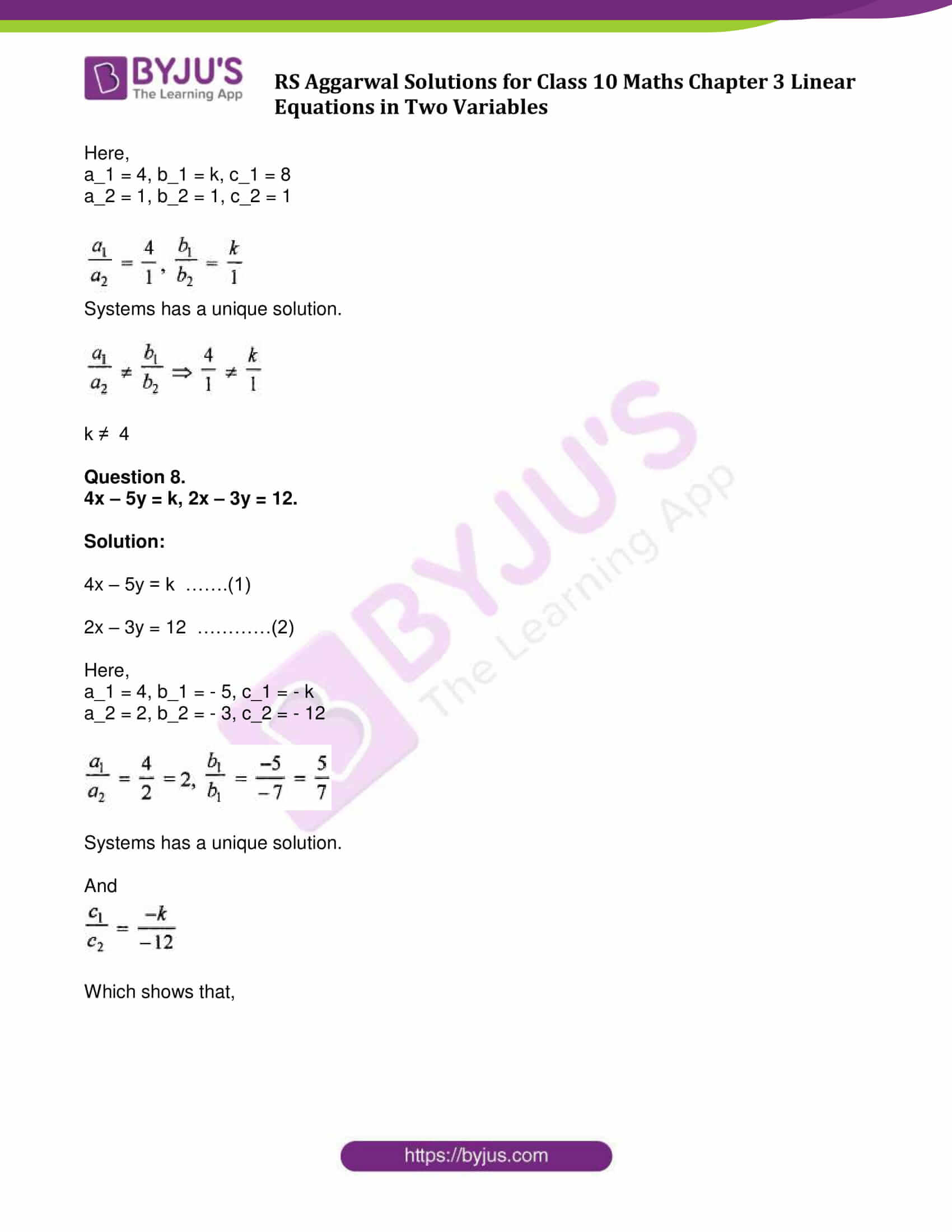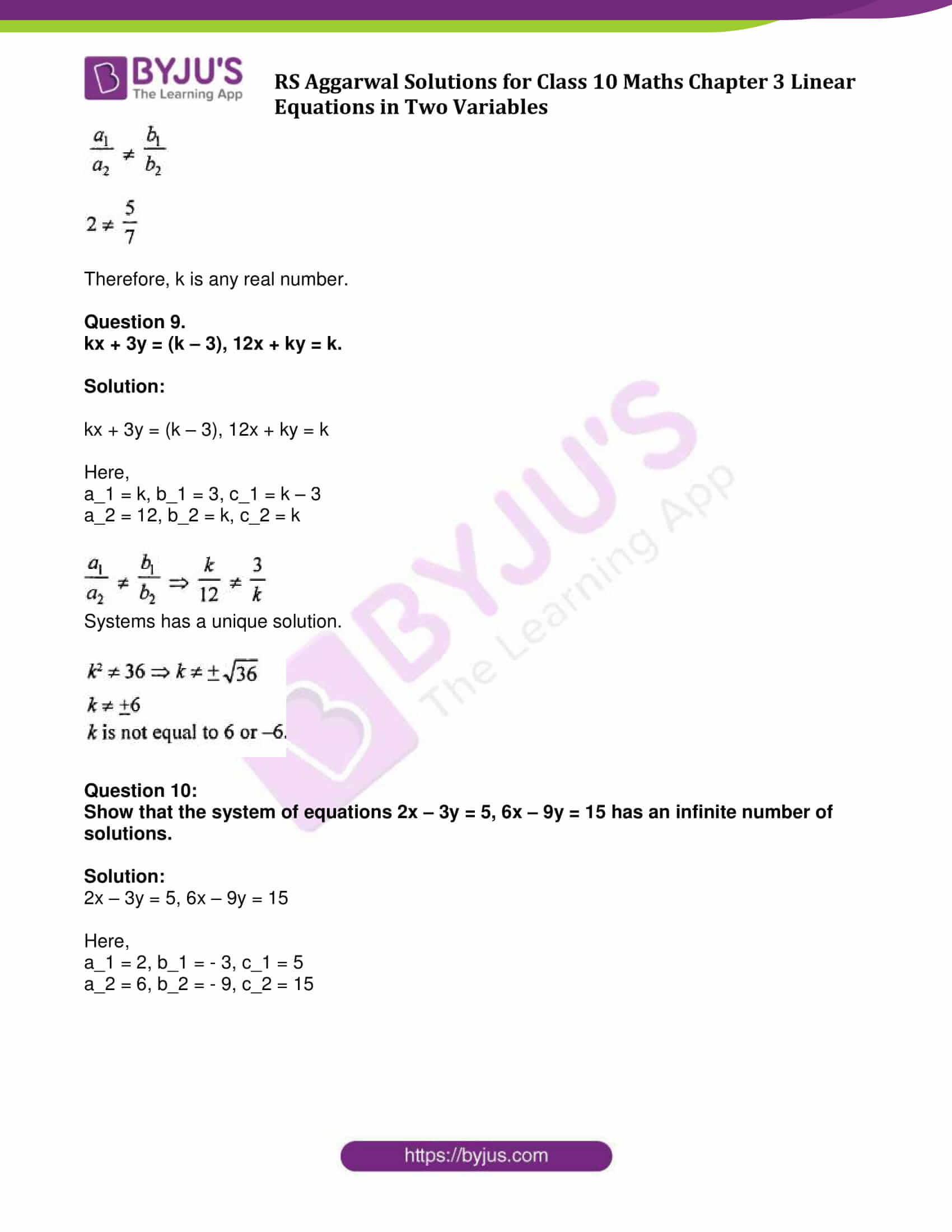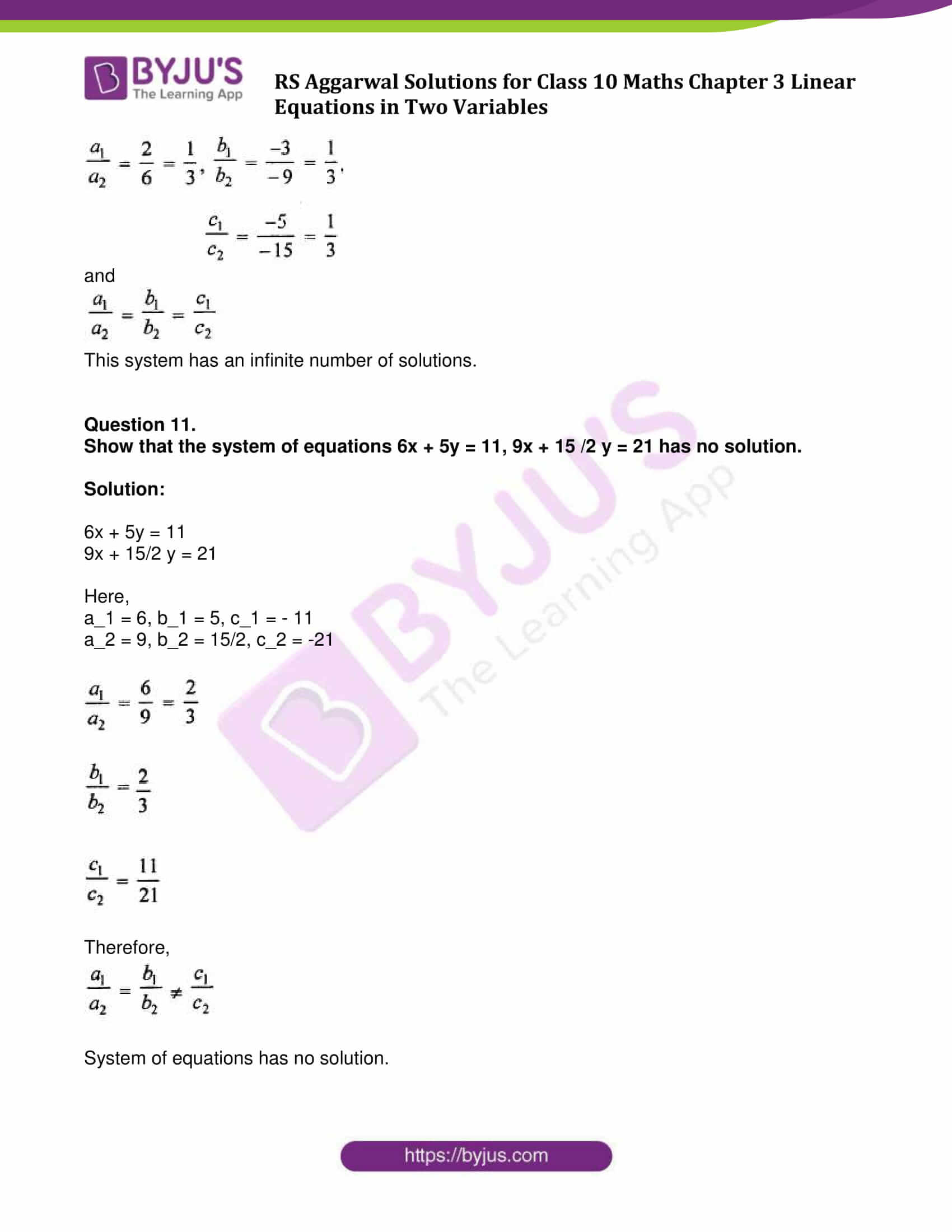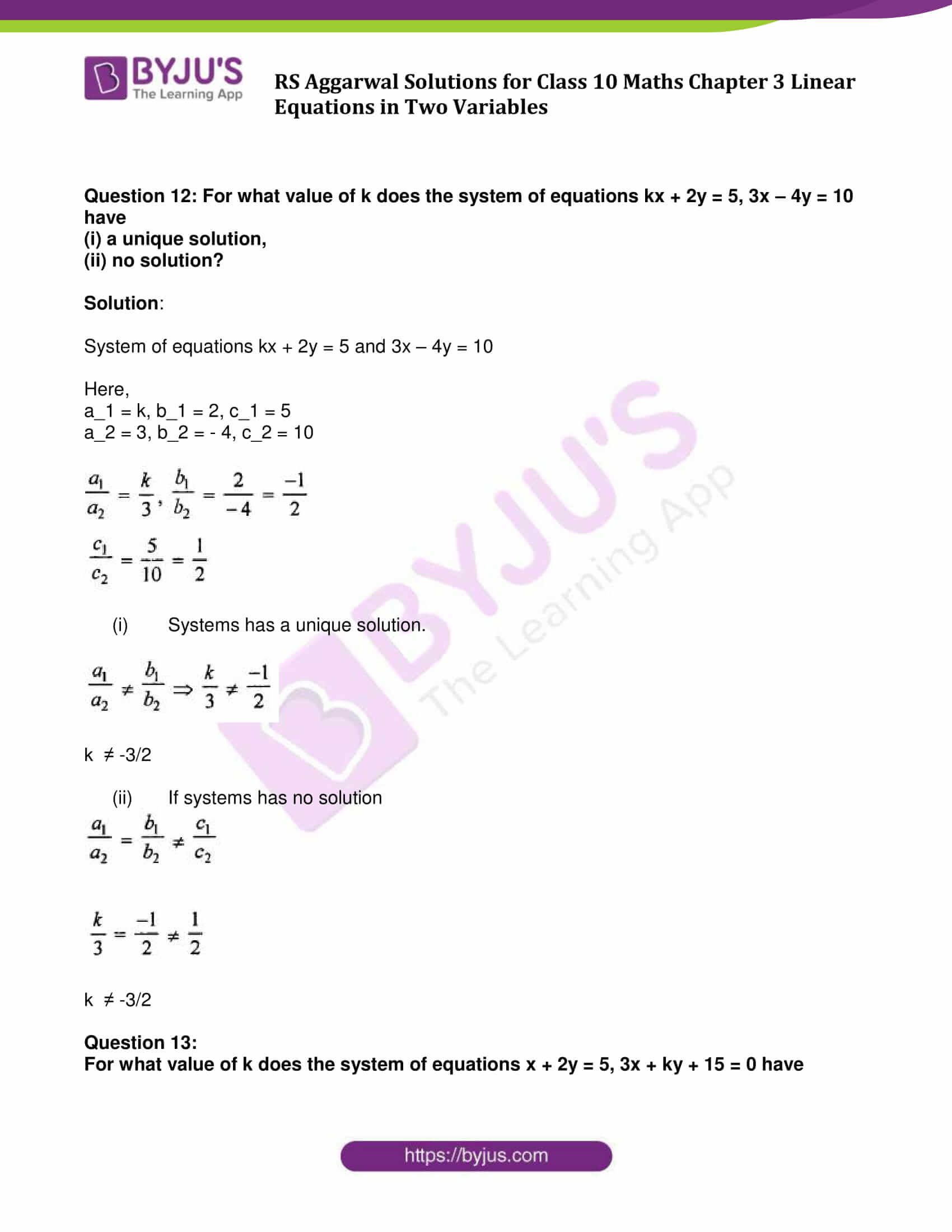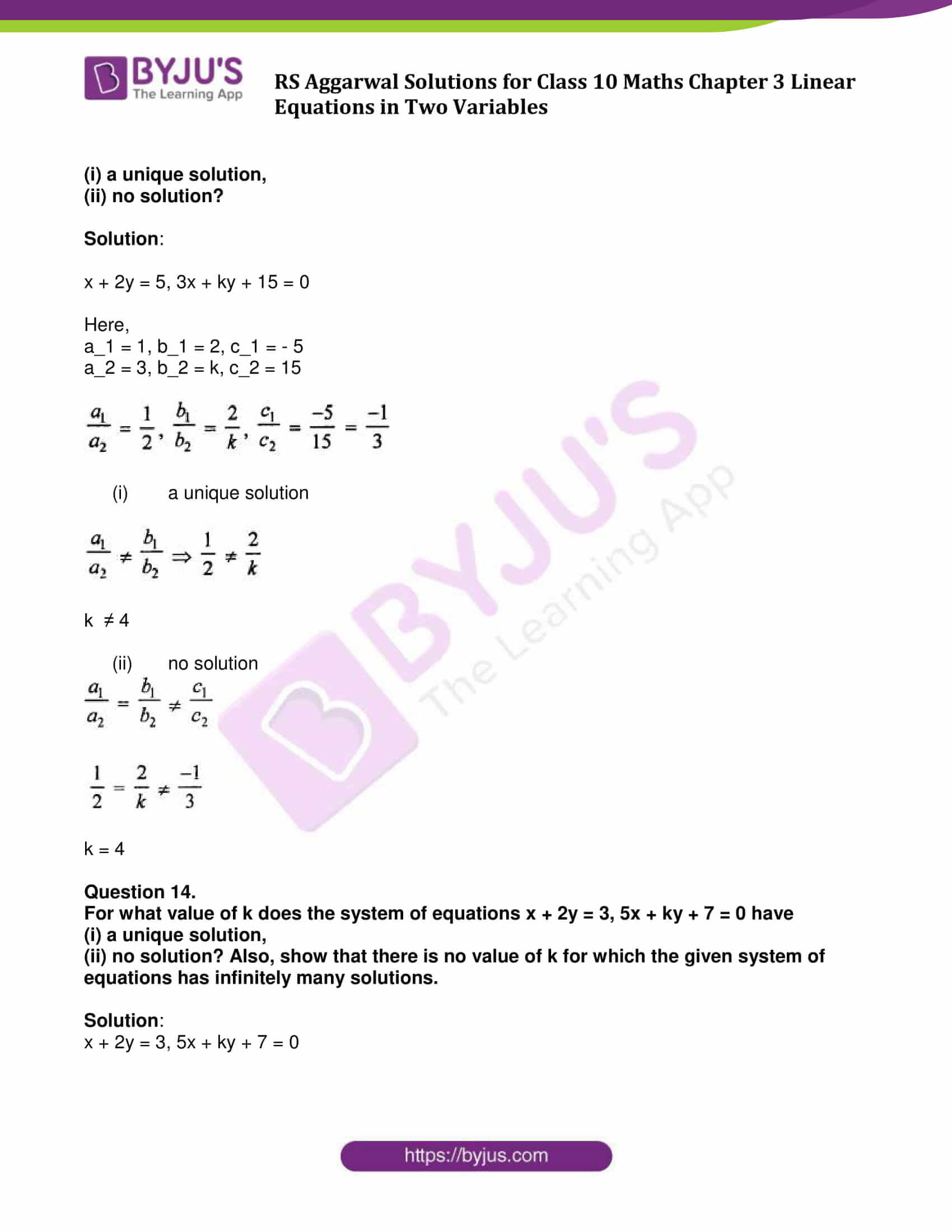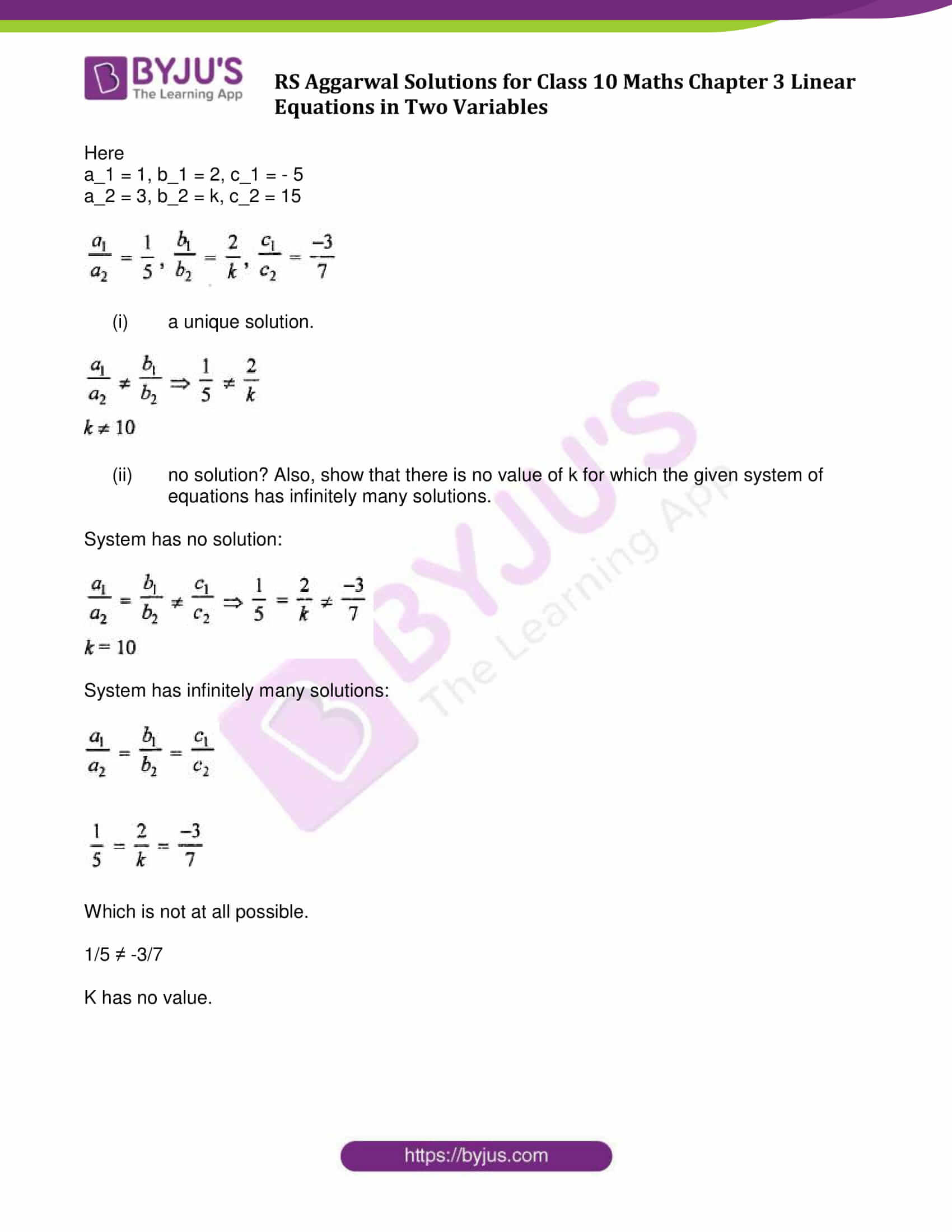## Access other exercise solutions of Class 10 Maths Chapter 3 Linear Equations in Two Variables

Exercise 3A Solutions: 29 Questions (Long Answers)

Exercise 3B Solutions: 20 Questions (Short Answers)

Exercise 3C Solutions: 13 Questions (Long Answers)

Exercise 3E Solutions: 20 Questions (Long Answers)

Exercise 3F Solutions: 10 Questions (6 Short Answers and 4 Long Answers)

## Exercise 3D Page No: 122

Show that each of the following systems of equations has a unique solution and solve it:

Question 1:

3x + 5y = 12, 5x + 3y = 4

Solution:

3x + 5y = 12 ……(1)

5x + 3y = 4 ……(2)

Here,

a_1 = 3, b_1 = 5, c_1 = 12

a_2 = 5, b_2 = 3, c_2 = 4

$\frac{a_{1}}{a_{2}} = \frac{3}{5} , \frac{b_{1}}{b_{2}} = \frac{5}{3}$

Which shows,

$\frac{a_{1}}{a_{2}} \neq \frac{b_{1}}{b_{2}}$

The system of equations have unique solution.

Solve the equations using substitution method:

From (1): x = (12-5y)/3

From (2): 5x + 3y – 4 = 0

5((12-5y)/3) + 3y – 4 = 0

60 – 25y + 9y = 12

60 – 16y = 12

16y = 48

y = 3

Now, substitute y in (1)

3x + 5(3) = 12

3x + 15 = 12

3x = 12 – 15

x = – 1

Answer: x = – 1 and y = 3

Question 2.

2x – 3y = 17, 4x + y = 13.

Solution:

2x – 3y = 17 ……..(1)

4x + y = 13 ………(2)

Here

a_1 = 2, b_1 = – 3, c_1 = 17

a_2 = 4, b_2 = 1, c_2 = 13

$\frac{a_{1}}{a_{2}} = \frac{2}{4} , \frac{b_{1}}{b_{2}} = \frac{-3}{1} = -3$

Which shows:

$\frac{a_{1}}{a_{2}} \neq \frac{b_{1}}{b_{2}}$

System has unique solutions.

Solve the equations using substitution method:

From (1): x = (17 + 3y)/2

Putting value of x in (2)

4((17 + 3y)/2) + y = 13

68 + 12y + 2y = 26

68 + 14y = 26

y = -3

Again, x = (17 + 3(-3))/2

2x = 8

x = 4

Answer: x = 4 and y = – 3

Question 3:

x/2 + y/2 = 3, x – 2y = 2

Solution:

2x + 3y = 18 …(1)

x – 2y = 2 …(2)

a_1 = 2, b_1 = 3, c_1 = 18

a_2 = 1, b_2 = – 2, c_2 = 2

$\frac{a_{1}}{a_{2}} = \frac{2}{1} = 2 , \frac{b_{1}}{b_{2}} = \frac{3}{-2}$

which shows ,

$\frac{a_{1}}{a_{2}} \neq \frac{b_{1}}{b_{2}}$

This system has a unique solution.

Solve the equations:

From (2), x = 2 + 2y

Substituting the value of x in (1),

2(2 + 2y) + 3y = 18

=> 4 + 4y + 3y = 18

=> 7y = 18 – 4 = 14

=> y = 2

and x = 2 + 2 x 2 = 2 + 4 = 6

Answer: x = 6 and y = 2

Find the value of k for which each of the following systems of equations has a unique solution:

Question 4:

2x + 3y – 5 = 0, kx – 6y – 8 = 0.

Solution:

2x + 3y – 5 = 0 ………….(1)

kx – 6y – 8 = 0 ………..(2)

a_1 = 2, b_1 = 3, c_1 = – 5

a_2 = k, b_2 = – 6, c_2 = – 8

$\frac{a_{1}}{a_{2}} = \frac{2}{k} , \frac{b_{1}}{b_{2}} = \frac{3}{-6}$

Which shows:

$\frac{a_{1}}{a_{2}} \neq \frac{b_{1}}{b_{2}}$

System has a unique solution.

Now, Find the value of k:

2/k ≠ 3/-6

k ≠ -4

Question 5.

x – ky = 2, 3x + 2y + 5 = 0.

Solution:

x – ky = 2 ….(1)

3x + 2y + 5 = 0 ….(2)

Here,

a_1 = 1, b_1 = – k, c_1 = – 2

a_2 = 3, b_2 = 2, c_2 = 5

$\frac{a_{1}}{a_{2}} = \frac{1}{3} = 2 , \frac{b_{1}}{b_{2}} = \frac{-k}{2} , \frac{c_{1}}{c_{2}} = \frac{2}{-5}$

Systems has a unique solution.

Now,

$\frac{a_{1}}{a_{2}} \neq \frac{b_{1}}{b_{2}} \Rightarrow \frac{1}{3} \neq \frac{k}{2}$

k ≠ 2/3

Question 6.

5x – 7y – 5 = 0, 2x + ky – 1 = 0.

Solution:

5x – 7y – 5 = 0 ….(1)

2x + ky – 1 = 0 …..(2)

Here,

a_1 = 5, b_1 = – 7, c_1 = – 5

a_2 = 2, b_2 = k, c_2 = – 1

$\frac{a_{1}}{a_{2}} = \frac{5}{2} , \frac{b_{1}}{b_{2}} = \frac{-7}{+k} = \frac{-7}{k}$

Systems has a unique solution.

$\frac{a_{1}}{a_{2}} \neq \frac{b_{1}}{b_{2}} \Rightarrow \frac{5}{2} \neq \frac{-7}{k}$

k ≠ -14/5

Question 7.

4x + ky + 8 = 0, x + y + 1 = 0.

Solution:

4x + ky + 8 = 0 ………(1)

x + y + 1 = 0 ………….(2)

Here,

a_1 = 4, b_1 = k, c_1 = 8

a_2 = 1, b_2 = 1, c_2 = 1

$\frac{a_{1}}{a_{2}} = \frac{4}{1} , \frac{b_{1}}{b_{2}} = \frac{k}{1}$

Systems has a unique solution.

$\frac{a_{1}}{a_{2}} \neq \frac{b_{1}}{b_{2}} \Rightarrow \frac{4}{1} \neq \frac{k}{1}$

k ≠ 4

Question 8.

4x – 5y = k, 2x – 3y = 12.

Solution:

4x – 5y = k …….(1)

2x – 3y = 12 …………(2)

Here,

a_1 = 4, b_1 = – 5, c_1 = – k

a_2 = 2, b_2 = – 3, c_2 = – 12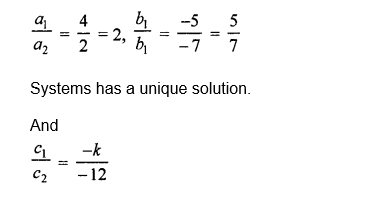Which shows that,

$\frac{a_{1}}{a_{2}} \neq \frac{b_{1}}{b_{2}}$

2 ≠ $\frac{5}{7}$

Therefore, k is any real number.

Question 9.

kx + 3y = (k – 3), 12x + ky = k.

Solution:

kx + 3y = (k – 3), 12x + ky = k

Here,

a_1 = k, b_1 = 3, c_1 = k – 3

a_2 = 12, b_2 = k, c_2 = k

$\frac{a_{1}}{a_{2}} \neq \frac{b_{1}}{b_{2}} \Rightarrow \frac{k}{12} \neq \frac{3}{4}$

Systems has a unique solution.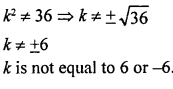Question 10:

Show that the system of equations 2x – 3y = 5, 6x – 9y = 15 has an infinite number of solutions.

Solution:

2x – 3y = 5, 6x – 9y = 15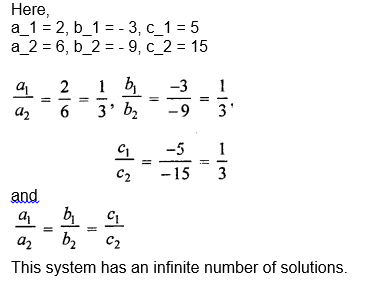Question 11.

Show that the system of equations 6x + 5y = 11, 9x + 15 /2 y = 21 has no solution.

Solution:

6x + 5y = 11

9x + 15/2 y = 21

Here,

a_1 = 6, b_1 = 5, c_1 = – 11

a_2 = 9, b_2 = 15/2, c_2 = -21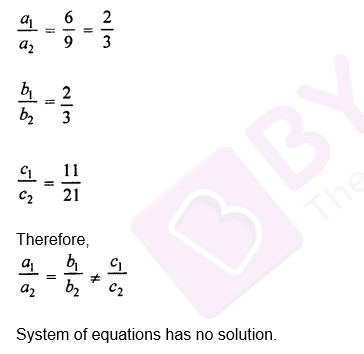Question 12: For what value of k does the system of equations kx + 2y = 5, 3x – 4y = 10 have

(i) a unique solution,

(ii) no solution?

Solution:

System of equations kx + 2y = 5 and 3x – 4y = 10

Here,

a_1 = k, b_1 = 2, c_1 = 5

a_2 = 3, b_2 = – 4, c_2 = 10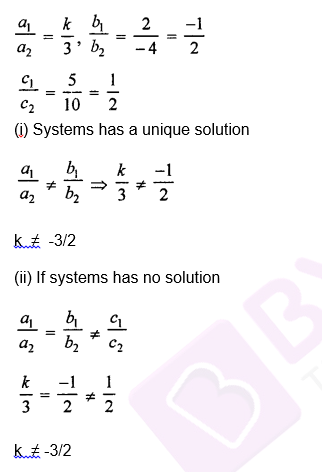Question 13:

For what value of k does the system of equations x + 2y = 5, 3x + ky + 15 = 0 have

(i) a unique solution,

(ii) no solution?

Solution:

x + 2y = 5, 3x + ky + 15 = 0

Here,

a_1 = 1, b_1 = 2, c_1 = – 5

a_2 = 3, b_2 = k, c_2 = 15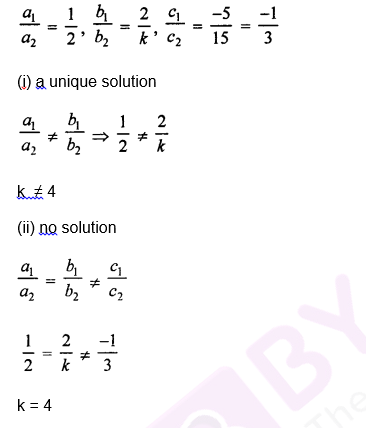Question 14.

For what value of k does the system of equations x + 2y = 3, 5x + ky + 7 = 0 have

(i) a unique solution,

(ii) no solution? Also, show that there is no value of k for which the given system of equations has infinitely many solutions.

Solution:

x + 2y = 3, 5x + ky + 7 = 0

Here

a_1 = 1, b_1 = 2, c_1 = – 5

a_2 = 3, b_2 = k, c_2 = 15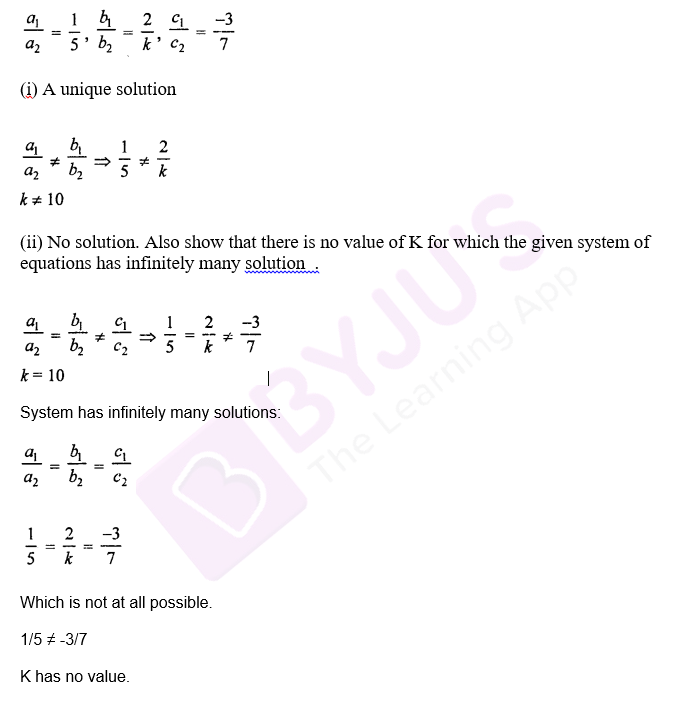## RS Aggarwal Solutions for Class 10 Maths Chapter 3 Linear Equations in Two Variables Exercise 3D

Class 10 Maths Chapter 3 Linear Equations in Two Variables Exercise 3d is based on the topic and subtopics:

• Conditions for solvability of linear equations
• Consistent and Inconsistent systems of linear equations
• Conditions for solvability of linear equations